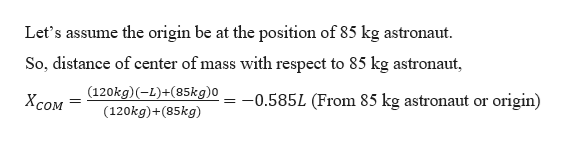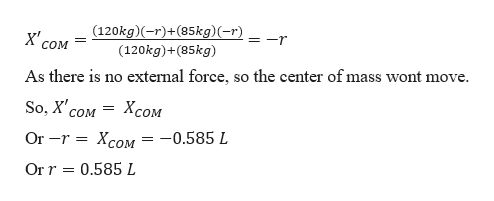Two space walkers, one with mass= 120 kg and the other one with mass = 85kg, hold on to the ends of a lightweight cable with length L=25m. The astronauts are originally at rest in deep space. If the astronauts then start pulling themselves towards each other along the cable until they neet, roughly how far will the less massive astronaut move? Express your answer as a fraction of L

Question

Two space walkers, one with mass= 120 kg and the other one with mass = 85kg, hold on to the ends of a lightweight cable with length L=25m. The astronauts are originally at rest in deep space. If the astronauts then start pulling themselves towards each other along the cable until they neet, roughly how far will the less massive astronaut move? Express your answer as a fraction of L

Step 1

Given:

Mass of 1st astronaut = 85 kg

Mass of 2nd astronaut = 120 kg

Distance between them, L = 25 m

Step 2

As both the astronauts are in deep space, so there is no external force acting on them. So, in the absence of external force, the center of mass of the system will remain stationary. Finding the initial position of center of mass of the system:help_outlineImage TranscriptioncloseLet's assume the origin be at the position of 85 kg astronaut. So, distance of center of mass with respect to 85 kg astronaut (120kg)(-L)+(85kg)0 0.585L (From 85 kg astronaut or origin) Хсом (120kg)+(85kg) fullscreen
Step 3

As the both astronauts move towards each-other, and finally meet. Let the both astronauts meet a...help_outlineImage Transcriptionclose(120kg)(-r)+(85kg)(-r) = -r COM (120kg)+(85kg) As there is no extemal force, so the center of mass wont move So, X сом Хсом Or -r 3D Xcом — — 0.585 L Or r 0.585 L fullscreen

Want to see the full answer?

See Solution

Want to see this answer and more?

Our solutions are written by experts, many with advanced degrees, and available 24/7

See Solution
Tagged in

Centre of Mass and Centre of Gravity